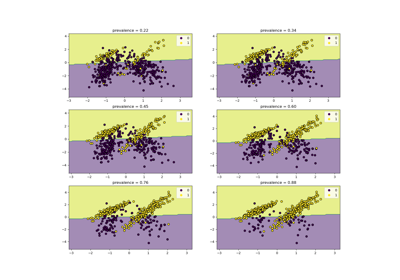# sklearn.dummy.DummyClassifier¶

class sklearn.dummy.DummyClassifier(*, strategy='prior', random_state=None, constant=None)[source]

DummyClassifier makes predictions that ignore the input features.

This classifier serves as a simple baseline to compare against other more complex classifiers.

The specific behavior of the baseline is selected with the strategy parameter.

All strategies make predictions that ignore the input feature values passed as the X argument to fit and predict. The predictions, however, typically depend on values observed in the y parameter passed to fit.

Note that the “stratified” and “uniform” strategies lead to non-deterministic predictions that can be rendered deterministic by setting the random_state parameter if needed. The other strategies are naturally deterministic and, once fit, always return the same constant prediction for any value of X.

Read more in the User Guide.

New in version 0.13.

Parameters:
strategy{“most_frequent”, “prior”, “stratified”, “uniform”, “constant”}, default=”prior”

Strategy to use to generate predictions.

• “most_frequent”: the predict method always returns the most frequent class label in the observed y argument passed to fit. The predict_proba method returns the matching one-hot encoded vector.

• “prior”: the predict method always returns the most frequent class label in the observed y argument passed to fit (like “most_frequent”). predict_proba always returns the empirical class distribution of y also known as the empirical class prior distribution.

• “stratified”: the predict_proba method randomly samples one-hot vectors from a multinomial distribution parametrized by the empirical class prior probabilities. The predict method returns the class label which got probability one in the one-hot vector of predict_proba. Each sampled row of both methods is therefore independent and identically distributed.

• “uniform”: generates predictions uniformly at random from the list of unique classes observed in y, i.e. each class has equal probability.

• “constant”: always predicts a constant label that is provided by the user. This is useful for metrics that evaluate a non-majority class.

Changed in version 0.24: The default value of strategy has changed to “prior” in version 0.24.

random_stateint, RandomState instance or None, default=None

Controls the randomness to generate the predictions when strategy='stratified' or strategy='uniform'. Pass an int for reproducible output across multiple function calls. See Glossary.

constantint or str or array-like of shape (n_outputs,), default=None

The explicit constant as predicted by the “constant” strategy. This parameter is useful only for the “constant” strategy.

Attributes:
classes_ndarray of shape (n_classes,) or list of such arrays

Unique class labels observed in y. For multi-output classification problems, this attribute is a list of arrays as each output has an independent set of possible classes.

n_classes_int or list of int

Number of label for each output.

class_prior_ndarray of shape (n_classes,) or list of such arrays

Frequency of each class observed in y. For multioutput classification problems, this is computed independently for each output.

n_outputs_int

Number of outputs.

sparse_output_bool

True if the array returned from predict is to be in sparse CSC format. Is automatically set to True if the input y is passed in sparse format.

DummyRegressor

Regressor that makes predictions using simple rules.

Examples

>>> import numpy as np
>>> from sklearn.dummy import DummyClassifier
>>> X = np.array([-1, 1, 1, 1])
>>> y = np.array([0, 1, 1, 1])
>>> dummy_clf = DummyClassifier(strategy="most_frequent")
>>> dummy_clf.fit(X, y)
DummyClassifier(strategy='most_frequent')
>>> dummy_clf.predict(X)
array([1, 1, 1, 1])
>>> dummy_clf.score(X, y)
0.75


Methods

 fit(X, y[, sample_weight]) Fit the baseline classifier. get_params([deep]) Get parameters for this estimator. Perform classification on test vectors X. Return log probability estimates for the test vectors X. Return probability estimates for the test vectors X. score(X, y[, sample_weight]) Return the mean accuracy on the given test data and labels. set_params(**params) Set the parameters of this estimator.
fit(X, y, sample_weight=None)[source]

Fit the baseline classifier.

Parameters:
Xarray-like of shape (n_samples, n_features)

Training data.

yarray-like of shape (n_samples,) or (n_samples, n_outputs)

Target values.

sample_weightarray-like of shape (n_samples,), default=None

Sample weights.

Returns:
selfobject

Returns the instance itself.

get_params(deep=True)[source]

Get parameters for this estimator.

Parameters:
deepbool, default=True

If True, will return the parameters for this estimator and contained subobjects that are estimators.

Returns:
paramsdict

Parameter names mapped to their values.

predict(X)[source]

Perform classification on test vectors X.

Parameters:
Xarray-like of shape (n_samples, n_features)

Test data.

Returns:
yarray-like of shape (n_samples,) or (n_samples, n_outputs)

Predicted target values for X.

predict_log_proba(X)[source]

Return log probability estimates for the test vectors X.

Parameters:
X{array-like, object with finite length or shape}

Training data.

Returns:
Pndarray of shape (n_samples, n_classes) or list of such arrays

Returns the log probability of the sample for each class in the model, where classes are ordered arithmetically for each output.

predict_proba(X)[source]

Return probability estimates for the test vectors X.

Parameters:
Xarray-like of shape (n_samples, n_features)

Test data.

Returns:
Pndarray of shape (n_samples, n_classes) or list of such arrays

Returns the probability of the sample for each class in the model, where classes are ordered arithmetically, for each output.

score(X, y, sample_weight=None)[source]

Return the mean accuracy on the given test data and labels.

In multi-label classification, this is the subset accuracy which is a harsh metric since you require for each sample that each label set be correctly predicted.

Parameters:
XNone or array-like of shape (n_samples, n_features)

Test samples. Passing None as test samples gives the same result as passing real test samples, since DummyClassifier operates independently of the sampled observations.

yarray-like of shape (n_samples,) or (n_samples, n_outputs)

True labels for X.

sample_weightarray-like of shape (n_samples,), default=None

Sample weights.

Returns:
scorefloat

Mean accuracy of self.predict(X) w.r.t. y.

set_params(**params)[source]

Set the parameters of this estimator.

The method works on simple estimators as well as on nested objects (such as Pipeline). The latter have parameters of the form <component>__<parameter> so that it’s possible to update each component of a nested object.

Parameters:
**paramsdict

Estimator parameters.

Returns:
selfestimator instance

Estimator instance.

## Examples using sklearn.dummy.DummyClassifier¶Class Likelihood Ratios to measure classification performance

Class Likelihood Ratios to measure classification performance